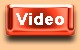You are here:GrammarArticlesDefinite ArticlesDative
 Definite Articles - DativeForms

The dative is the third of the German cases. In other languages, as in English, French or Spanish, we sometimes have to use prepositions if we have a dative object which corresponds to the indirect object.

 Here are the definite articles in the third case (dative):
 Singular Forms Definite Article (with Noun) Phonetic Script English Translation masculine dem (Mann m) [de:m] (to) the (man) feminine der (Frau f) [de:ɐ] (to) the (woman) neuter dem (Kind nt) [de:m] (to) the (child) Plural Forms Definite Article (with Noun) Phonetic Script English Translation masculine (Männern m pl) (to) the (men) feminine den (Frauen f pl) [de:n] (to) the (women) neuter (Kindern nt pl) (to) the (children)

As you can see, there is only one plural form of the definite article ("den") for every noun, no matter if it is masculine, feminine or neuter.

Dative after certain verbs

The dative case is used for the indirect object. In German it is called dative object.

Here is an example sentence:

 German Phonetic Script English Ich vertraue dem Jungen m. [ɪç fɛɐ'trauə de:m 'jʊŋən] I trust the boy.

While there is only one object form in English, we use either the dative or the accusative case in German. It depends on the verb which case you have to use. The explanation is that some German verbs are followed by the dative case but most of them are followed by the accusative case. Simply look it up in a dictionary if you have a certain verb and do not know the case which it is used with. You can also use an online dictionary and enter the respective verb. If you enter the verb "vertrauen", there is written: jdm (jemandem) vertrauen. "jemandem" always means dative.

 German Phonetic Script English jemandem (jdm) vertrauen ['je:mandəm fɛɐ'trauən] to trust someone

If you ask for the dative object (or indirect object) of the example sentence, you ask: Whom (do I trust)? The answer in this case would be: The boy.

While "Ich" is the subject of the sentence in the nominative case because it is is the acting part of the sentence to which the predicate refers to (I am doing something), "Jungen" is the noun in the dative case and thus forms the dative object (=indirect object) together with the article. Very often a person receives something. In this case, the boy receives my trust.

Prepositions with dative

After certain prepositions we must also use the dative case: ab (from/as of), aus (out of/from), bei (with/at), bis zu (up to), gegenüber (opposite), mit (with), nach (after), seit (since/for), von (of/from), zu (to). Here is one example:

 German Phonetic Script English Sie kommt vom (= von dem) Bahnhof m. [zi: kɔmt fɔm 'ba:nho:f] She is coming from the station.

"Bahnhof" is a masculine (m) noun (der Bahnhof). Here we have to put it in the dative case.

Please take into consideration that there is a melting between certain prepositions and some of the definite articles:

 Case + Definite Article Preposition Definite Article Melting Dative + Masculine/Neuter Definite Article an + dem = am Dative + Masculine/Neuter Definite Article bei + dem = beim Dative + Masculine/Neuter Definite Article in + dem = im Dative + Masculine/Neuter Definite Article von + dem = vom Dative + Masculine/Neuter Definite Article zu + dem = zum Dative + Feminine Definite Article zu + der = zur

Wechselpräpositionen

After the following prepositions (Wechselpräpositionen) you have to use the dative case if you ask: Where?: an (at/on), auf (on), hinter (behind), in (in), neben (next to), über (over, above), unter (under/below), vor (in front of), zwischen (between). Here is one example:

 German Phonetic Script English Wir sind in der Stadt f. [vi:ə zɪnt ɪn de:ɐ ʃtat] We are in town.

Where are we? We are in town. But be careful: If there is a verb which involves a movement and you would ask "Where to?", then you have to use the accusative case. Read more about the use of the accusative if you click on the respective button above.

There are also adjectives which are used with certain prepositions. After these adjectives we must also use the dative case. Here is one example:

 German Phonetic Script English Er ist mit der Lösung f einverstanden. [e:ɐ ɪst mɪt de:ɐ 'lø:zʊŋ 'ainfɛɐʃtandən] He agrees with the solution. mit etwas einverstanden sein [mɪt 'ɛtvas 'ainfɛɐʃtandən zain] to agree with something

If you are insecure about the preposition and the case, simply look it up in an online dictionary.

Idiomatic expressions

The dative is also used with certain set expressions. Here is one example:

 German Phonetic Script English Dem Jungen m ist kalt. [de:m 'jʊŋən ɪst kalt] The boy is cold.